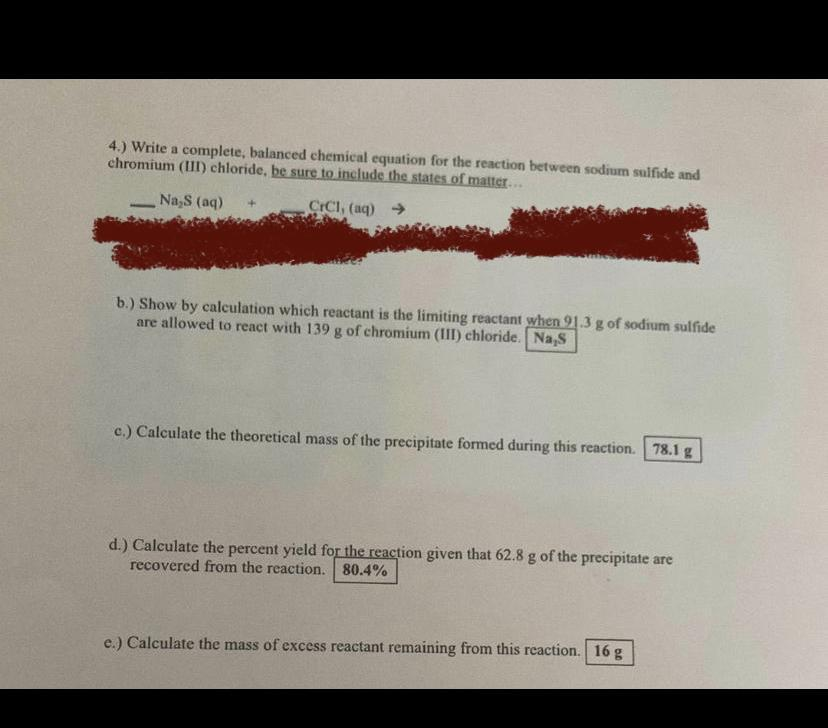Question:

# 4.) Write a complete, balanced chemical equation for the reaction between sodium sulfide and chromium(III) chloride, be sure to4.) Write a complete, balanced chemical equation for the reaction between sodium sulfide and chromium(III) chloride, be sure to include the states of matter... Na S (aq) CICI, (aq) → b.) Show by calculation which reactant is the limiting reactant when 21.3 g of sodium sulfide are allowed to react with 139 g of chromium (III) chloride. Na,s c.) Calculate the theoretical mass of the precipitate formed during this reaction. 78.1 g d.) Calculate the percent yield for the reaction given that 62.8 g of the precipitate are recovered from the reaction. 80.4% e.) Calculate the mass of excess reactant remaining from this reaction. 16 g